# Solution for submission 132083

A detailed solution for submission 132083 submitted for challenge IIT-M RL-ASSIGNMENT-2-GRIDWORLD

# What is the notebook about?¶

## Problem - Gridworld Environment Algorithms¶

This problem deals with a grid world and stochastic actions. The tasks you have to do are:

• Implement Policy Iteration
• Implement Value Iteration
• Implement TD lamdda
• Visualize the results
• Explain the results

## How to use this notebook? 📝¶

• This is a shared template and any edits you make here will not be saved.You should make a copy in your own drive. Click the "File" menu (top-left), then "Save a Copy in Drive". You will be working in your copy however you like.

• Update the config parameters. You can define the common variables here

Variable Description
AICROWD_DATASET_PATH Path to the file containing test data. This should be an absolute path.
AICROWD_RESULTS_DIR Path to write the output to.
AICROWD_ASSETS_DIR In case your notebook needs additional files (like model weights, etc.,), you can add them to a directory and specify the path to the directory here (please specify relative path). The contents of this directory will be sent to AIcrowd for evaluation.
AICROWD_API_KEY In order to submit your code to AIcrowd, you need to provide your account's API key. This key is available at https://www.aicrowd.com/participants/me

# Setup AIcrowd Utilities 🛠¶

We use this to bundle the files for submission and create a submission on AIcrowd. Do not edit this block.

In :
!pip install aicrowd-cli > /dev/null

ERROR: google-colab 1.0.0 has requirement requests~=2.23.0, but you'll have requests 2.25.1 which is incompatible.
ERROR: datascience 0.10.6 has requirement folium==0.2.1, but you'll have folium 0.8.3 which is incompatible.


# AIcrowd Runtime Configuration 🧷¶

Get login API key from https://www.aicrowd.com/participants/me

In :
import os

AICROWD_DATASET_PATH = os.getenv("DATASET_PATH", os.getcwd()+"/a5562c7d-55f0-4d06-841c-110655bb04ec_a2_gridworld_inputs.zip")
AICROWD_RESULTS_DIR = os.getenv("OUTPUTS_DIR", "results")

In :


API Key valid
Saved API Key successfully!
a5562c7d-55f0-4d06-841c-110655bb04ec_a2_gridworld_inputs.zip: 100% 14.2k/14.2k [00:00<00:00, 563kB/s]

In :
!unzip -q $AICROWD_DATASET_PATH  In : DATASET_DIR = 'inputs/'  # GridWorld Environment¶ Read the code for the environment thoroughly Do not edit the code for the environment In : import numpy as np class GridEnv_HW2: def __init__(self, goal_location, action_stochasticity, non_terminal_reward, terminal_reward, grey_in, brown_in, grey_out, brown_out ): # Do not edit this section self.action_stochasticity = action_stochasticity self.non_terminal_reward = non_terminal_reward self.terminal_reward = terminal_reward self.grid_size = [10, 10] # Index of the actions self.actions = {'N': (1, 0), 'E': (0,1), 'S': (-1,0), 'W': (0,-1)} self.perpendicular_order = ['N', 'E', 'S', 'W'] l = ['normal' for _ in range(self.grid_size) ] self.grid = np.array([l for _ in range(self.grid_size) ], dtype=object) self.grid[goal_location, goal_location] = 'goal' self.goal_location = goal_location for gi in grey_in: self.grid[gi,gi] = 'grey_in' for bi in brown_in: self.grid[bi, bi] = 'brown_in' for go in grey_out: self.grid[go, go] = 'grey_out' for bo in brown_out: self.grid[bo, bo] = 'brown_out' self.grey_outs = grey_out self.brown_outs = brown_out def _out_of_grid(self, state): if state < 0 or state < 0: return True elif state > self.grid_size - 1: return True elif state > self.grid_size - 1: return True else: return False def _grid_state(self, state): return self.grid[state, state] def get_transition_probabilites_and_reward(self, state, action): """ Returns the probabiltity of all possible transitions for the given action in the form: A list of tuples of (next_state, probability, reward) Note that based on number of state and action there can be many different next states Unless the state is All the probabilities of next states should add up to 1 """ grid_state = self._grid_state(state) if grid_state == 'goal': return [(self.goal_location, 1.0, 0.0)] elif grid_state == 'grey_in': npr = [] for go in self.grey_outs: npr.append((go, 1/len(self.grey_outs), self.non_terminal_reward)) return npr elif grid_state == 'brown_in': npr = [] for bo in self.brown_outs: npr.append((bo, 1/len(self.brown_outs), self.non_terminal_reward)) return npr direction = self.actions.get(action, None) if direction is None: raise ValueError("Invalid action %s , please select among" % action, list(self.actions.keys())) dir_index = self.perpendicular_order.index(action) wrap_acts = self.perpendicular_order[dir_index:] + self.perpendicular_order[:dir_index] next_state_probs = {} for prob, a in zip(self.action_stochasticity, wrap_acts): d = self.actions[a] next_state = (state + d), (state + d) if self._out_of_grid(next_state): next_state = state next_state_probs.setdefault(next_state, 0.0) next_state_probs[next_state] += prob npr = [] for ns, prob in next_state_probs.items(): next_grid_state = self._grid_state(ns) reward = self.terminal_reward if next_grid_state == 'goal' else self.non_terminal_reward npr.append((ns, prob, reward)) return npr def step(self, state, action): npr = self.get_transition_probabilites_and_reward(state, action) probs = [t for t in npr] sampled_idx = np.random.choice(range(len(npr)), p=probs) sampled_npr = npr[sampled_idx] next_state = sampled_npr reward = sampled_npr is_terminal = next_state == tuple(self.goal_location) return next_state, reward, is_terminal  ## Example environment¶ This has the same setup as the pdf, do not edit the settings In : def get_base_kwargs(): goal_location = (9,9) action_stochasticity = [0.8, 0.2/3, 0.2/3, 0.2/3] grey_out = [(3,2), (4,2), (5,2), (6,2)] brown_in = [(9,7)] grey_in = [(0,0)] brown_out = [(1,7)] non_terminal_reward = 0 terminal_reward = 10 base_kwargs = {"goal_location": goal_location, "action_stochasticity": action_stochasticity, "brown_in": brown_in, "grey_in": grey_in, "brown_out": brown_out, "non_terminal_reward": non_terminal_reward, "terminal_reward": terminal_reward, "grey_out": grey_out,} return base_kwargs base_kwargs = get_base_kwargs()  ## Task 2.1 - Value Iteration¶ Run value iteration on the environment and generate the policy and expected reward In : def value_iteration(env, gamma): # Initial Values values = np.zeros((10, 10)) # Initial policy policy = np.empty((10, 10), object) policy[:] = 'N' # Make all the policy values as 'N' # Begin code here value_history = [values.copy()] states = [(i,j) for i in range(env.grid_size) for j in range(env.grid_size)] epsilon = 1e-8 delta = epsilon while delta >= epsilon: delta = 0 H = np.zeros((10, 10)) - float('inf') for s in states: for action in env.perpendicular_order: npr = env.get_transition_probabilites_and_reward(s, action) c = 0 for next_state, probability, reward in npr: c += probability * (reward + gamma*values[next_state]) H[s] = max(H[s], c) delta = max(delta, abs(values[s] - H[s])) values = H.copy() value_history.append(values.copy()) for s in states: C = -float('inf') A = env.perpendicular_order for action in env.perpendicular_order: npr = env.get_transition_probabilites_and_reward(s, action) c = 0 for next_state, probability, reward in npr: c += probability * (reward + gamma*values[next_state]) if c > C: C = c A = action policy[s] = A # Put your extra information needed for plots etc in this dictionary extra_info = {"All Values:": value_history} # End code # Do not change the number of output values return {"Values": values, "Policy": policy}, extra_info  In : env = GridEnv_HW2(**base_kwargs) res, extra_info = value_iteration(env, 0.7) # The rounding off is just for making print statement cleaner print(np.flipud(np.round(res['Values'], decimals=2))) print(np.flipud(res['Policy']))  [[0.1 0.15 0.24 0.37 0.56 0.86 1.29 0.12 8.68 0. ] [0.13 0.2 0.31 0.5 0.81 1.31 2.12 3.43 5.75 8.95] [0.1 0.16 0.25 0.39 0.62 0.97 1.52 2.38 3.7 5.61] [0.07 0.11 0.17 0.26 0.41 0.64 0.99 1.54 2.38 3.52] [0.05 0.07 0.11 0.17 0.27 0.41 0.64 0.99 1.53 2.21] [0.03 0.05 0.07 0.11 0.17 0.27 0.41 0.64 0.98 1.39] [0.02 0.03 0.05 0.07 0.11 0.17 0.27 0.41 0.63 0.87] [0.03 0.02 0.03 0.05 0.07 0.11 0.17 0.27 0.4 0.55] [0.04 0.03 0.02 0.03 0.05 0.07 0.11 0.17 0.26 0.35] [0.07 0.04 0.03 0.02 0.03 0.05 0.07 0.11 0.17 0.22]] [['E' 'E' 'E' 'E' 'E' 'S' 'S' 'N' 'E' 'N'] ['E' 'E' 'E' 'E' 'E' 'E' 'E' 'E' 'E' 'N'] ['E' 'E' 'E' 'E' 'E' 'E' 'E' 'E' 'N' 'N'] ['E' 'E' 'E' 'E' 'E' 'E' 'E' 'E' 'N' 'N'] ['N' 'E' 'E' 'E' 'E' 'E' 'E' 'N' 'N' 'N'] ['N' 'E' 'E' 'E' 'E' 'E' 'N' 'N' 'N' 'N'] ['N' 'N' 'E' 'E' 'E' 'N' 'N' 'N' 'N' 'N'] ['S' 'N' 'E' 'E' 'E' 'N' 'N' 'N' 'N' 'N'] ['S' 'S' 'E' 'E' 'E' 'E' 'N' 'N' 'N' 'N'] ['N' 'W' 'W' 'E' 'E' 'E' 'E' 'N' 'N' 'N']]  ## Task 2.2 - Policy Iteration¶ Run policy iteration on the environment and generate the policy and expected reward In : def policy_iteration(env, gamma): # Initial Values values = np.zeros((10, 10)) # Initial policy policy = np.empty((10, 10), object) policy[:] = 'N' # Make all the policy values as 'N' # Begin code here reduced_value_history = [values.copy()] value_history = [values.copy()] states = [(i,j) for i in range(env.grid_size) for j in range(env.grid_size)] epsilon = 1e-8 done = False while not done: delta = epsilon while delta >= epsilon: delta = 0 for s in states: j = values[s] npr = env.get_transition_probabilites_and_reward(s, policy[s]) c = 0 for next_state, probability, reward in npr: c += probability * (reward + gamma*values[next_state]) values[s] = c delta = max(delta, abs(j - values[s])) value_history.append(values.copy()) reduced_value_history.append(values.copy()) done = True for s in states: b = policy[s] C = -float('inf') A = env.perpendicular_order for action in env.perpendicular_order: npr = env.get_transition_probabilites_and_reward(s, action) c = 0 for next_state, probability, reward in npr: c += probability * (reward + gamma*values[next_state]) if c > C: C = c A = action policy[s] = A if b != policy[s]: done = False # Put your extra information needed for plots etc in this dictionary extra_info = {"All Values:": value_history, "Reduced Values:": reduced_value_history} # End code # Do not change the number of output values return {"Values": values, "Policy": policy}, extra_info  In : env = GridEnv_HW2(**base_kwargs) res, extra_info = policy_iteration(env, 0.7) # The rounding off is just for making print statement cleaner print(np.flipud(np.round(res['Values'], decimals=2))) print(np.flipud(res['Policy']))  [[0.1 0.15 0.24 0.37 0.56 0.86 1.29 0.12 8.68 0. ] [0.13 0.2 0.31 0.5 0.81 1.31 2.12 3.43 5.75 8.95] [0.1 0.16 0.25 0.39 0.62 0.97 1.52 2.38 3.7 5.61] [0.07 0.11 0.17 0.26 0.41 0.64 0.99 1.54 2.38 3.52] [0.05 0.07 0.11 0.17 0.27 0.41 0.64 0.99 1.53 2.21] [0.03 0.05 0.07 0.11 0.17 0.27 0.41 0.64 0.98 1.39] [0.02 0.03 0.05 0.07 0.11 0.17 0.27 0.41 0.63 0.87] [0.03 0.02 0.03 0.05 0.07 0.11 0.17 0.27 0.4 0.55] [0.04 0.03 0.02 0.03 0.05 0.07 0.11 0.17 0.26 0.35] [0.07 0.04 0.03 0.02 0.03 0.05 0.07 0.11 0.17 0.22]] [['E' 'E' 'E' 'E' 'E' 'S' 'S' 'N' 'E' 'N'] ['E' 'E' 'E' 'E' 'E' 'E' 'E' 'E' 'E' 'N'] ['E' 'E' 'E' 'E' 'E' 'E' 'E' 'E' 'N' 'N'] ['E' 'E' 'E' 'E' 'E' 'E' 'E' 'E' 'N' 'N'] ['N' 'E' 'E' 'E' 'E' 'E' 'E' 'N' 'N' 'N'] ['N' 'E' 'E' 'E' 'E' 'E' 'N' 'N' 'N' 'N'] ['N' 'N' 'E' 'E' 'E' 'N' 'N' 'N' 'N' 'N'] ['S' 'N' 'E' 'E' 'E' 'N' 'N' 'N' 'N' 'N'] ['S' 'S' 'E' 'E' 'E' 'E' 'N' 'N' 'N' 'N'] ['N' 'W' 'W' 'E' 'E' 'E' 'E' 'N' 'N' 'N']]  # Task 2.3 - TD Lambda¶ Use the heuristic policy and implement TD lambda to find values on the gridworld In : # The policy mentioned in the pdf to be used for TD lambda, do not modify this def heuristic_policy(env, state): goal = env.goal_location dx = goal - state dy = goal - state if abs(dx) >= abs(dy): direction = (np.sign(dx), 0) else: direction = (0, np.sign(dy)) for action, dir_val in env.actions.items(): if dir_val == direction: target_action = action break return target_action  In : def td_lambda(env, lamda, seeds): alpha = 0.5 gamma = 0.7 N = len(seeds) # Usage of input_policy # heuristic_policy(env, state) -> action example_action = heuristic_policy(env, (1,2)) # Returns 'N' if goal is (9,9) # Example of env.step # env.step(state, action) -> Returns next_state, reward, is_terminal # Initial values values = np.zeros((10, 10)) es = np.zeros((10,10)) value_history = [values.copy()] for episode_idx in range(N): # Do not change this else the results will not match due to environment stochas np.random.seed(seeds[episode_idx]) grey_in_loc = np.where(env.grid == 'grey_in') state = grey_in_loc, grey_in_loc done = False while not done: action = heuristic_policy(env, state) ns, rew, is_terminal = env.step(state, action) # env.step is already taken inside the loop for you, # Don't use env.step anywhere else in your code # Begin code here delta = rew + gamma*values[ns] - values[state] es[state] = es[state] + 1 for i in range(env.grid_size): for j in range(env.grid_size): values[i,j] = values[i,j] + alpha * delta * es[i,j] es[i,j] = gamma * lamda * es[i,j] state = ns value_history.append(values.copy()) if state == env.goal_location: done=True # Put your extra information needed for plots etc in this dictionary extra_info = {"All Values:": value_history} # End code # Do not change the number of output values return {"Values": values}, extra_info  In : env = GridEnv_HW2(**base_kwargs) res, extra_info = td_lambda(env, lamda=0.5, seeds=np.arange(1000)) # The rounding off is just for making print statement cleaner print(np.flipud(np.round(res['Values'], decimals=2)))  [[ 0. 0. 0.01 0.02 0.03 0.06 0.08 0.11 10. 0. ] [ 0. 0. 0.04 0.18 0.91 1.4 1.28 4.85 6.98 9.92] [ 0. 0.05 0.24 0.42 0.63 0.71 2.08 3.28 3.8 5.89] [ 0.02 0.08 0.21 0.31 0.48 0.82 1.29 1.66 2.2 3.39] [ 0.02 0.07 0.08 0.11 0.25 0.4 0.52 0.96 1.62 1.44] [ 0.02 0.04 0.05 0.1 0.16 0.23 0.38 0.57 1.08 0.97] [ 0.01 0.03 0.03 0.05 0.08 0.17 0.2 0.35 0.58 0.26] [ 0. 0.01 0.03 0.03 0.07 0.1 0.15 0.23 0.18 0.18] [ 0. 0. 0.01 0.01 0.03 0.03 0.1 0.15 0.16 0. ] [ 0.12 0. 0. 0. 0. 0.01 0.01 0.11 0.13 0. ]]  # Task 2.4 - TD Lamdba for multiple values of$\lambda$¶ Ideally this code should run as is In : # This cell is only for your subjective evaluation results, display the results as asked in the pdf # You can change it as you require, this code should run TD lamdba by default for different values of lambda lamda_values = np.arange(0, 100+5, 5)/100 td_lamda_results = {} extra_info = {} for lamda in lamda_values: env = GridEnv_HW2(**base_kwargs) td_lamda_results[lamda], extra_info[lamda] = td_lambda(env, lamda, seeds=np.arange(1000))  # Generate Results ✅¶ In : def get_results(kwargs): gridenv = GridEnv_HW2(**kwargs) policy_iteration_results = policy_iteration(gridenv, 0.7) value_iteration_results = value_iteration(gridenv, 0.7) td_lambda_results = td_lambda(env, 0.5, np.arange(1000)) final_results = {} final_results["policy_iteration"] = policy_iteration_results final_results["value_iteration"] = value_iteration_results final_results["td_lambda"] = td_lambda_results return final_results  In : # Do not edit this cell, generate results with it as is if not os.path.exists(AICROWD_RESULTS_DIR): os.mkdir(AICROWD_RESULTS_DIR) for params_file in os.listdir(DATASET_DIR): kwargs = np.load(os.path.join(DATASET_DIR, params_file), allow_pickle=True).item() results = get_results(kwargs) idx = params_file.split('_')[-1][:-4] np.save(os.path.join(AICROWD_RESULTS_DIR, 'results_' + idx), results)  # Check your score on the public data¶ This scores is not your final score, and it doesn't use the marks weightages. This is only for your reference of how arrays are matched and with what tolerance. In : # Check your score on the given test cases (There are more private test cases not provided) target_folder = 'targets' result_folder = AICROWD_RESULTS_DIR def check_algo_match(results, targets): if 'Policy' in results: policy_match = results['Policy'] == targets['Policy'] else: policy_match = True # Reference https://numpy.org/doc/stable/reference/generated/numpy.allclose.html rewards_match = np.allclose(results['Values'], targets['Values'], rtol=3) equal = rewards_match and policy_match return equal def check_score(target_folder, result_folder): match = [] for out_file in os.listdir(result_folder): res_file = os.path.join(result_folder, out_file) results = np.load(res_file, allow_pickle=True).item() idx = out_file.split('_')[-1][:-4] # Extract the file number target_file = os.path.join(target_folder, f"targets_{idx}.npy") targets = np.load(target_file, allow_pickle=True).item() algo_match = [] for k in targets: algo_results = results[k] algo_targets = targets[k] algo_match.append(check_algo_match(algo_results, algo_targets)) match.append(np.mean(algo_match)) return np.mean(match) if os.path.exists(target_folder): print("Shared data Score (normalized to 1):", check_score(target_folder, result_folder))  Shared data Score (normalized to 1): 1.0  /usr/local/lib/python3.7/dist-packages/numpy/core/_asarray.py:136: VisibleDeprecationWarning: Creating an ndarray from ragged nested sequences (which is a list-or-tuple of lists-or-tuples-or ndarrays with different lengths or shapes) is deprecated. If you meant to do this, you must specify 'dtype=object' when creating the ndarray return array(a, dtype, copy=False, order=order, subok=True)  ## Display Results of TD lambda¶ Display Results of TD lambda with lambda values from 0 to 1 with steps of 0.05 Add code/text as required # Subjective questions¶ ## 2.a Value Iteration vs Policy Iteration¶ 1. Compare value iteration and policy iteration for states Brown in, Brown Out, Grey out and Grey In 2. Which one converges faster and why In : import matplotlib.pyplot as plt env = GridEnv_HW2(**base_kwargs) val_res, val_extra_info = value_iteration(env, 0.7) pol_res, pol_extra_info = policy_iteration(env, 0.7) bin = [(i,j) for i in range(env.grid.shape) for j in range(env.grid.shape) if env.grid[i,j] == 'brown_in'] bout = [(i,j) for i in range(env.grid.shape) for j in range(env.grid.shape) if env.grid[i,j] == 'brown_out'] gin = [(i,j) for i in range(env.grid.shape) for j in range(env.grid.shape) if env.grid[i,j] == 'grey_in'] gout = [(i,j) for i in range(env.grid.shape) for j in range(env.grid.shape) if env.grid[i,j] == 'grey_out'] #print(bin, "\n", bout, "\n", gin, "\n", gout) val_bin = [vals[bi] for vals in val_extra_info["All Values:"] for bi in bin] val_bout = [vals[bi] for vals in val_extra_info["All Values:"] for bi in bout] val_gin = [vals[bi] for vals in val_extra_info["All Values:"] for bi in gin] val_gout = [[vals[bi] for vals in val_extra_info["All Values:"]] for bi in gout] pol_bin = [vals[bi] for vals in pol_extra_info["All Values:"] for bi in bin] pol_bout = [vals[bi] for vals in pol_extra_info["All Values:"] for bi in bout] pol_gin = [vals[bi] for vals in pol_extra_info["All Values:"] for bi in gin] pol_gout = [[vals[bi] for vals in pol_extra_info["All Values:"]] for bi in gout] pol_bin2 = [vals[bi] for vals in pol_extra_info["Reduced Values:"] for bi in bin] pol_bout2 = [vals[bi] for vals in pol_extra_info["Reduced Values:"] for bi in bout] pol_gin2 = [vals[bi] for vals in pol_extra_info["Reduced Values:"] for bi in gin] pol_gout2 = [[vals[bi] for vals in pol_extra_info["Reduced Values:"]] for bi in gout] ######################### bin ############################## plt.figure(figsize=(12, 8), dpi=80) plt.title("J('brown_in') vs number of iterations") plt.xlabel("number of iterations") plt.ylabel("J('brown_in')") plt.plot(val_bin, label="value iteration") plt.plot(pol_bin2, label="policy iteration") plt.scatter(range(len(val_bin)), val_bin) plt.scatter(range(len(pol_bin2)), pol_bin2) plt.legend() ######################### bout ############################## plt.figure(figsize=(12, 8), dpi=80) plt.title("J('brown_out') vs number of iterations") plt.xlabel("number of iterations") plt.ylabel("J('brown_out')") plt.plot(val_bout, label="value iteration") plt.plot(pol_bout2, label="policy iteration") plt.scatter(range(len(val_bout)), val_bout) plt.scatter(range(len(pol_bout2)), pol_bout2) plt.legend() ######################### gin ############################## plt.figure(figsize=(12, 8), dpi=80) plt.title("J('grey_in') vs number of iterations") plt.xlabel("number of iterations") plt.ylabel("J('grey_in')") plt.plot(val_gin, label="value iteration") plt.plot(pol_gin2, label="policy iteration") plt.scatter(range(len(val_gin)), val_gin) plt.scatter(range(len(pol_gin2)), pol_gin2) plt.legend() ######################### gout ############################## for i in range(len(gout)): plt.figure(figsize=(12, 8), dpi=80) plt.title("J('grey_out %d') vs number of iterations" % i) plt.xlabel("number of iterations") plt.ylabel("J('grey_out %d')" % i) plt.plot(val_gout[i], label="value iteration") plt.plot(pol_gout2[i], label="policy iteration") plt.scatter(range(len(val_gout[i])), val_gout[i]) plt.scatter(range(len(pol_gout2[i])), pol_gout2[i]) plt.legend()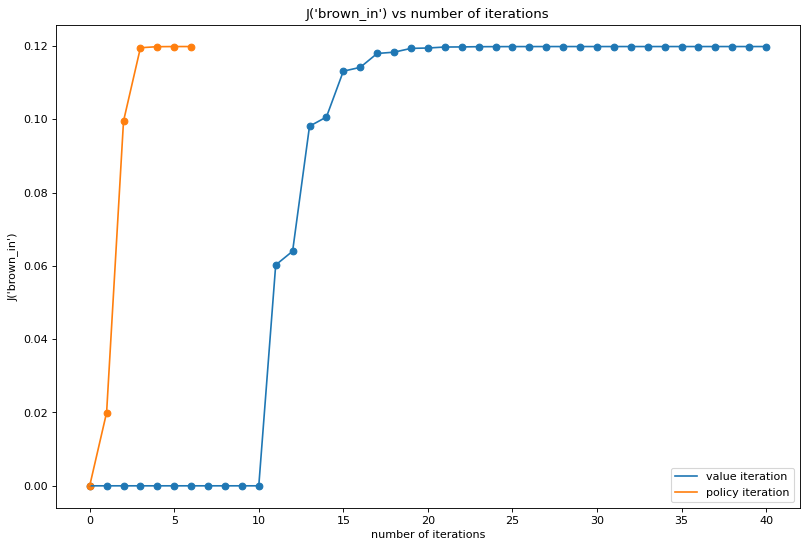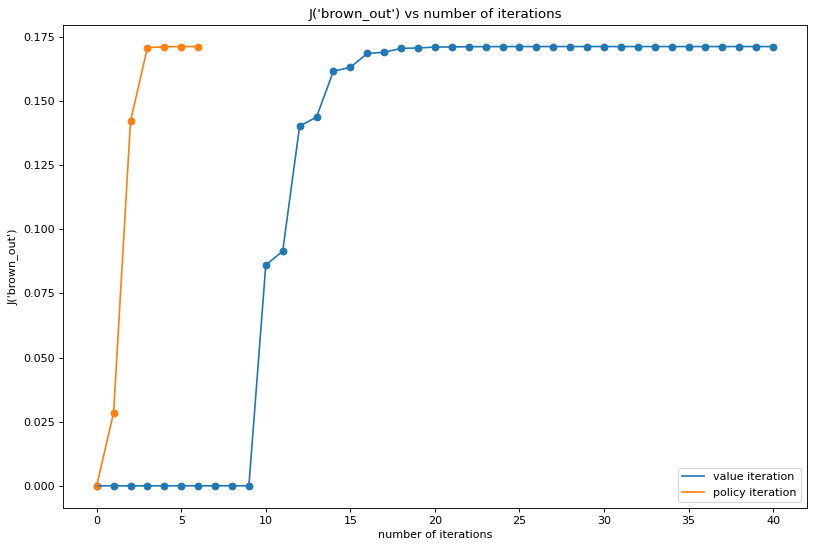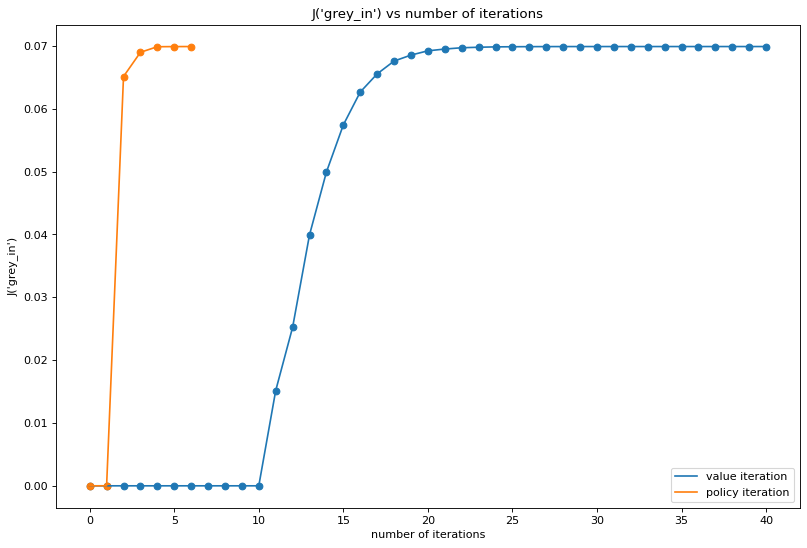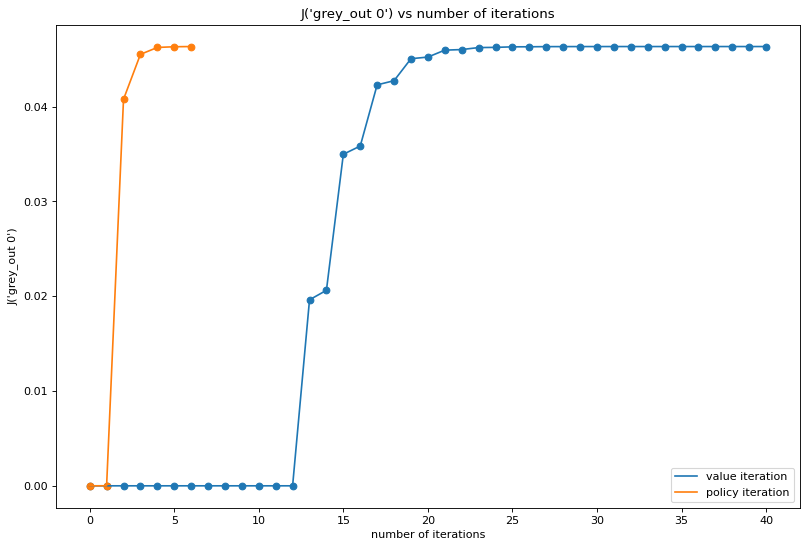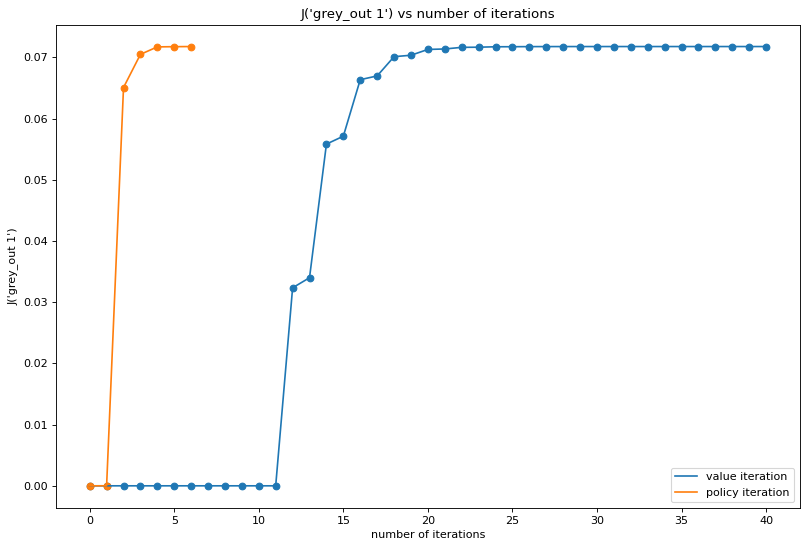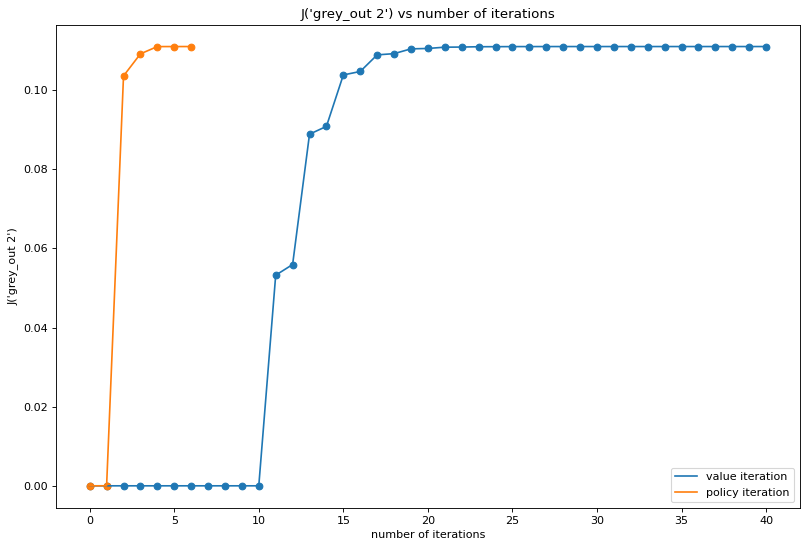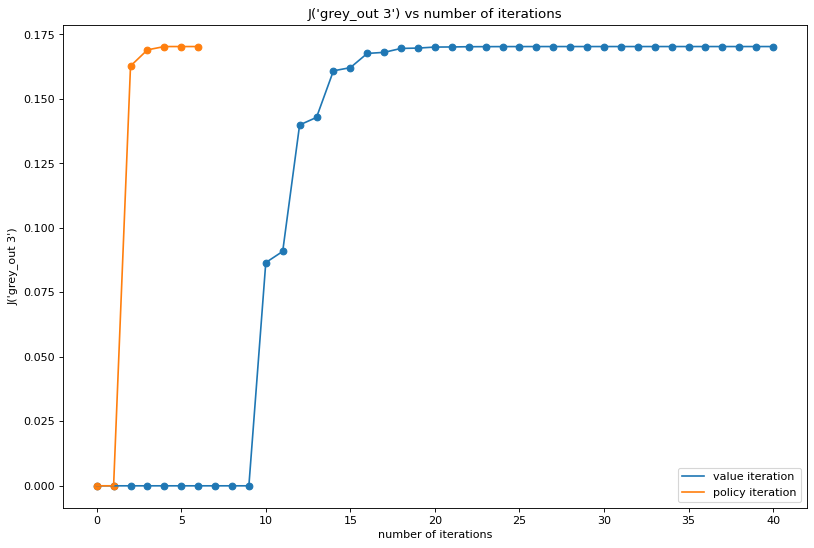Answers: 1. As is apparent from the plots above, policy iteration rapidly increaseses J until it appears to saturate in the last 3-4 iterations before policy iteration terminates whereas value iteration increases J more gradually only saturates it for the the last ~25 iterations. In value iteration the J values do not increase by large amounts in all of the first 15 iterations. In some iterations, only small increases happen. However, in policy iteration, the J values increase by large amounts in every (non-saturating) iteration. 2. Policy iteration converges in fewer iterations because in every step of policy iteration, a better policy is found whereas it may not be the case that policy changes between two subsequent iterations of value iteration. ## 2.b How changing$\lambda$affecting TD Lambda¶ In : td_bin = {l: td_lamda_results[l]["Values"][bi] for bi in bin for l in lamda_values} td_bout = {l: td_lamda_results[l]["Values"][bi] for bi in bout for l in lamda_values} td_gin = {l: td_lamda_results[l]["Values"][bi] for bi in gin for l in lamda_values} td_gout1 = {l: td_lamda_results[l]["Values"][bi] for bi in [gout] for l in lamda_values} vbin = pol_res['Values'][bin] vbout = pol_res['Values'][bout] vgin = pol_res['Values'][gin] vgout = pol_res['Values'][gout] plt.figure(figsize=(12, 8), dpi=80) plt.title("J vs lambda in TD(lambda)") plt.xlabel("lamdba") plt.ylabel("J") plt.plot(list(td_bin.keys()), list(td_bin.values()), label="J('brown_in')") plt.scatter(list(td_bin.keys()), list(td_bin.values())) plt.plot(list(td_bout.keys()), list(td_bout.values()), label="J('brown_out')") plt.scatter(list(td_bout.keys()), list(td_bout.values())) plt.plot(list(td_gin.keys()), list(td_gin.values()), label="J('grey_in')") plt.scatter(list(td_gin.keys()), list(td_gin.values())) plt.plot(list(td_gout1.keys()), list(td_gout1.values()), label="J('grey_out 0')") plt.scatter(list(td_gout1.keys()), list(td_gout1.values())) #plt.plot([0,1], [vbin, vbin], linestyle = 'dashed', label="J*('brown_in')", color="blue", linewidth=1) #plt.plot([0,1], [vbout, vbout], linestyle = 'dashed', label="J*('brown_out')", color="orange", linewidth=1) #plt.plot([0,1], [vgin, vgin], linestyle = 'dashed', label="J*('grey_in')", color="green", linewidth=1) #plt.plot([0,1], [vgout, vgout], linestyle = 'dashed', label="J*('grey_out')", color="red", linewidth=1) #plt.plot([, keys[-1]], [vi_results[0.9]['Expected Reward']['B'], vi_results[0.9]['Expected Reward']['B']], linestyle = 'dashed', label="J*(B)", color="orange") #plt.plot([keys, keys[-1]], [vi_results[0.9]['Expected Reward']['C'], vi_results[0.9]['Expected Reward']['C']], linestyle = 'dashed', label="J*(C)", color="green") plt.legend()  Out: <matplotlib.legend.Legend at 0x7fd8baa75d10>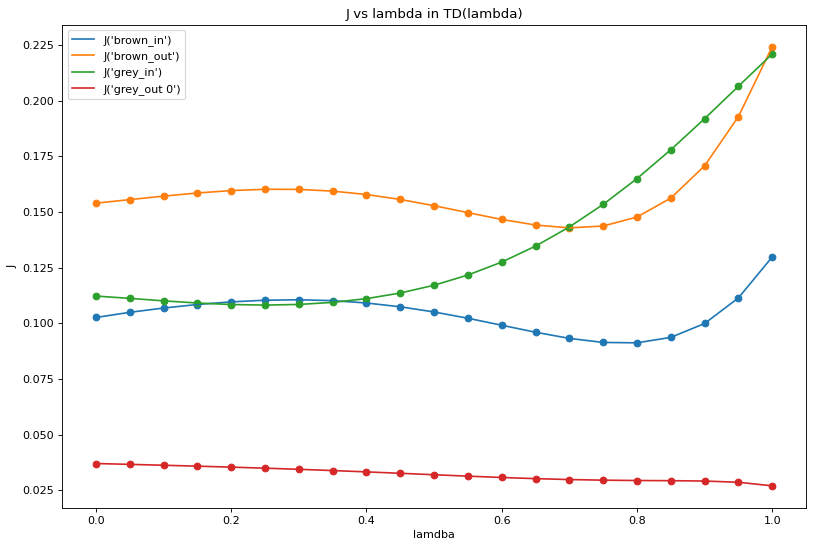Answer: The effects of changing lambda and running TD(lambda) on this environment are plotted above. I have plotted the curves obtained for brown_in, brown_out and grey_in cells and one of the grey_out cells. The curve obtained for brown_in and borwn_out increases, decreased and then increases whereas the curve for grey_in increases monotonically in this range and the curve for grey_out_0 seems to decrease monotonically. The general pattern we see is that at lambda = 1, they veer away from the true values of J* (obtained by value iteration. And get closest to it somwhere around lambda = 0.5. This is because of high variance at lambda = 1 and high bias at lambda = 0. lambda = ~0.5 proves to be a satisfactory choice in this tradeoff. ## 2.c Policy iteration error curve¶ Plot error curve of$J_i$vs iteration$i$for policy iteration In : #print(pol_res['Values']) #print(pol_extra_info["Reduced Values:"]) pol_errors = [np.sum(np.square(pol_extra_info['Reduced Values:'][i] - val_res['Values'])/(env.grid_size * env.grid_size))**0.5 for i in range(len(pol_extra_info["Reduced Values:"]))] plt.figure(figsize=(12, 8), dpi=80) plt.title("Error in J_i vs number of iterations for policy iteration") plt.xlabel("number of iterations i") plt.ylabel("J* - J_i") plt.plot(pol_errors, label="error") plt.scatter(range(len(pol_errors)), pol_errors) plt.legend()  Out: <matplotlib.legend.Legend at 0x7fd8baa6c210>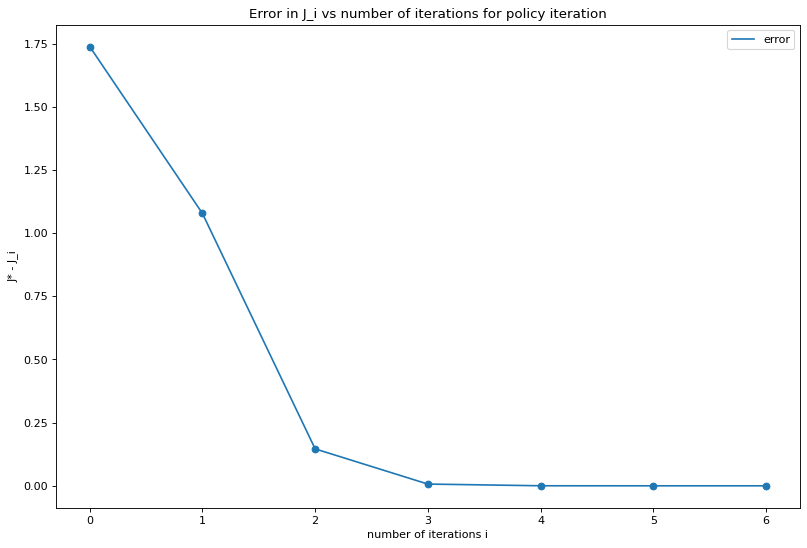## 2.d TD Lamdba error curve¶ Plot error curve of$J_i$vs iteration$i$for TD Lambda for$\lambda = [0, 0.25, 0.5, 0.75, 1]$In : lamda_values = [0, 0.25, 0.5, 0.75, 1] td_errors = [np.sum(np.square(td_lamda_results[l]['Values'] - val_res['Values'])/(env.grid_size * env.grid_size))**0.5 for l in lamda_values] plt.figure(figsize=(12, 8), dpi=80) plt.title("Error in J vs lambda for TD(lambda)") plt.xlabel("lambda") plt.ylabel("error") plt.plot(lamda_values, td_errors, label="error") plt.scatter(lamda_values, td_errors) plt.legend()  Out: <matplotlib.legend.Legend at 0x7fd8ba977850>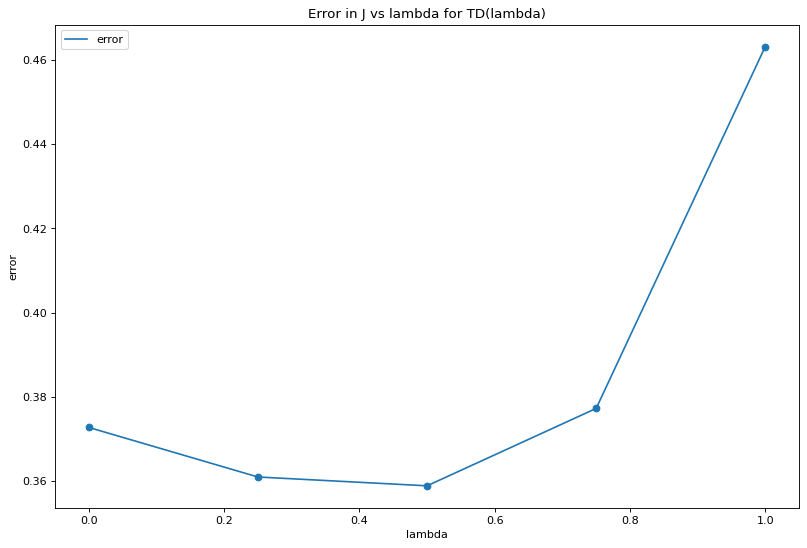# Submit to AIcrowd 🚀¶ In [ ]: !DATASET_PATH=$AICROWD_DATASET_PATH aicrowd notebook submit --no-verify -c iit-m-rl-assignment-2-gridworld -a assets

WARNING: No assets directory at assets... Creating one...
No jupyter lab module found. Using jupyter notebook.
Using notebook: /content/Copy%20of%20IITM_Assignment_2_Gridworld_Release.ipynb for submission...